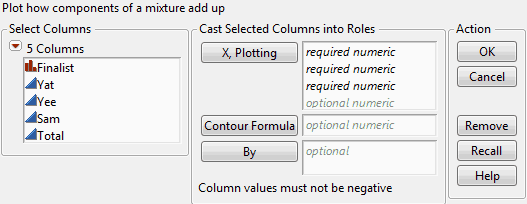Essential Graphing > Ternary Plots > Launch the Ternary Plot Platform
Publication date: 04/12/2021

# Launch the Ternary Plot Platform

Launch Ternary Plot by selecting Graph > Ternary Plot.

Figure 11.5 The Ternary Plot Launch WindowX, Plotting

Assign three columns to generate a ternary plot.

If you assign more than 3 variables to the X, Plotting role, a matrix of ternary plots is created. A separate variable is assigned to the first two axes of a plot, with the third axis being the sum of the other variables. If necessary, the variables are scaled so they sum to 1.

Contour Formula

To plot contours of a response surface, assign a column containing a formula to the Contour Formula role. If you have variables in a Contour formula that are not listed as X, Plotting variables, JMP appends sliders below the plot so that the values can be interactively adjusted. See Example Using a Contour Function.

By

This option produces a separate graph for each level of the By variable.

After you click OK, the Ternary Plot window appears. See The Ternary Plot.

Want more information? Have questions? Get answers in the JMP User Community (community.jmp.com).